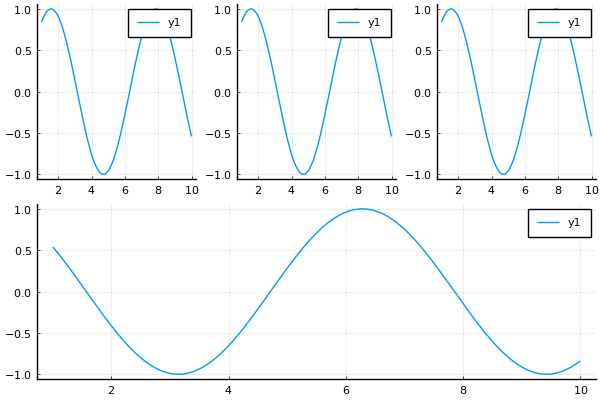# Plot layout with parametrized number of rows/columns

Hi all

I would like to incrementally build a plot from different subplots using `layout`. The issue I’m struggling with is that the number of subplots is a parameter/variable. So I would like to build a list of subplots, then pass this on to `plot` with a certain layout that is built using `grid`. Code example (that doesn’t work but I don’t seem to figure out to get it working):

Nplots = 3
l = @layout [Plots.grid(1, Nplots); b]

p = empty()

x = range(1,10,1001)

for i in range(1, Nplots)
append!(p, plot(x, sin.(x)))
end

q = plot(x, cos.(x))

plot(p…, q, layout = l)

What am I missing here? Is there a “standard” way to incrementally build up a plot from subplots where the number of subplots is variable?

Hi! Not sure about Plots.jl, but Makie.jl has a very nice layouting system, both flexible and powerful, and you can build the layout step by step.

The layout tutorial will give you an idea of what can be done.

For your example a starting point could be:

``````using CairoMakie

Nplots = 3
x = range(1,10,1001)

fig = Figure(resolution=(1000, 300))
for i in 1:Nplots
lines(fig[1,i], x, sin.(x))
end
fig
``````

and later you can do

``````lines(fig[1,end+1], x, cos.(x))
fig
``````

and you can also append to the left or top:

``````lines(fig[begin-1, 3:4], x, tan.(x))  # this is fig[0, 3:4] :-)
fig
``````

Thanks but I would like to avoid Makie for the moment simply because it uses Float32’s under the hood and I’ve run into multiple issues with precision loss (in log plots)…

As far as I can see, the problem is not in the layout. What you have to do is this:

• `p = []` instead of `p = empty()`
• `push!(p, plot(x, sin.(x)))` instead of `append!(p, plot(x, sin.(x)))`

After these changes the code worked correctly and produced the following pictureAlso the ‘standard’ way to define ranges is `1:Nplots`, not `range(1, Nplots)`.

Thanks for the solution. Any insight on what the background of my mistakes is? `empty()` vs `[]` and `push!` vs `append!`? Just so I understand and not blindly copy/paste?
I think you formatted the example code using a markdown quote instead of a code block, so the `empty` line shows a checkbox instead of `empty([ ])`. Then when we copy the code we get just `empty()`, which is not valid Julia.
Anyway `p = empty([ ])` is not wrong but a useless complication of `p = []` (writing `[]` already makes an empty vector, no need to make another one by calling `empty`.
We write `push!(x, yi)` to append one value `yi` to `x`, and `append!(x, y)` to append a collection of values. So `append!(p, plot(x, sin.(x)))` will take the result of `plot`, and take what is inside (a subplot) to append to the `p` vector. In the end you get a vector of subplots instead of a vector of plots.